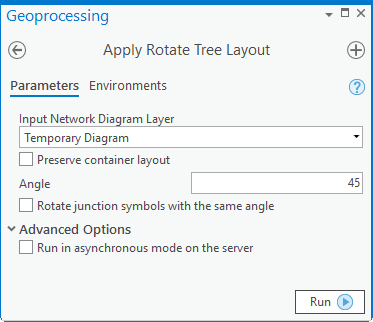# Rotate Tree layout reference

Rotate Tree is a schematic layout algorithm that applies to any type of diagram.

This layout algorithm rotates the tree or trees related to the pivot junctions currently set up in the input network diagram layer according to the specified angle.

For each processed diagram junction, it also optionally adds the specified angle value to the system field whose label is "Element rotation". This allows you to vary the diagram junction symbols by rotation using this field value so the symbols of the diagram junctions belonging to the tree or trees are also rotated according to the same rotation angle.

The Rotate Tree algorithm requires at least one pivot junction, such as a junction used as the center point around which the related graph will be rotated. The Rotate Tree layout also takes into account any barriers in the input diagram layer to prevent the algorithm from executing beyond the barriers.

The image below shows a sample diagram:

A pivot junction is set on the sample diagram:

A barrier is positioned on the sample diagram:

The next image shows the sample diagram after applying the Rotate Tree layout:

If the Rotate Tree layout requires at least one pivot junction to process, it can run without setting any barrier, such as in the sample below:

In addition, several pivot junctions can be set on the diagrams, such as in the following example:

## Apply the Rotate Tree layout on an active diagram map view

When applying this layout on a network diagram, the following prerequisites must be met:

• Since this operation is transactional, edits must be saved before it is run.
• The input network diagram layer to which the layout applies must be from either a utility network or trace network in a file geodatabase, or a network diagram service. When working with a utility network or a trace network in an enterprise geodatabase, the input network diagram layer must be from a service
• At least one junction must be set as a pivot junction in the input network diagram.

To apply the Rotate Tree layout on an active diagram map view, do one of the following:

• On the Network Diagram tab, click the drop-down arrow on the Diagram Layouts button in the Layout group, and click the Rotate Tree layout item in the gallery.
• Load the Apply Rotate Tree Layout tool in the Geoprocessing pane.

Then, adjust the Rotate Tree layout parameters and click Run.

##### Note:

To apply the layout on a subset of features in the network diagram, use one of the Select Features tools (for example, Select By Rectangle, Select By Polygon, and so on) and select the diagram features before running.

## Rotate Tree layout parametersThe sections below clarify the main Rotate Tree diagram layout parameters.

### Preserve container layout

Most of the layout algorithms work with the Preserve container layout option. This option allows controlling the algorithm execution so it executes either on the top graph of the diagramPreserve container layout checked, or on both content and noncontent features in the diagram—Preserve container layout unchecked.

### Angle

This parameter is used to set the angle in degrees that will be used to rotate the tree:

### Rotate junction symbols with the same angle

Each diagram junction is stored in its junction feature class with a rotation system field which label is "Element rotation" and whose value is zero by default. The Rotate junction symbols with the same angle option specifies whether the specified Angle value must be added to the current value of the rotation field for each processed diagram junction.

• Checked—The specified Angle value will add to the current value of the rotation field for each processed diagram junction.
• Unchecked—The rotation field value will not change during the layout process. This is the default.

##### Tip:

The Rotate Tree layout is the only layout that edits the rotation field when it executes with the Rotate junction symbols with the same angle option checked. Then, to get your diagram junctions to automatically display with rotated symbols according to this rotation value at the end of the layout execution as in the graphic below, you must set up the diagram layer definition accordingly.